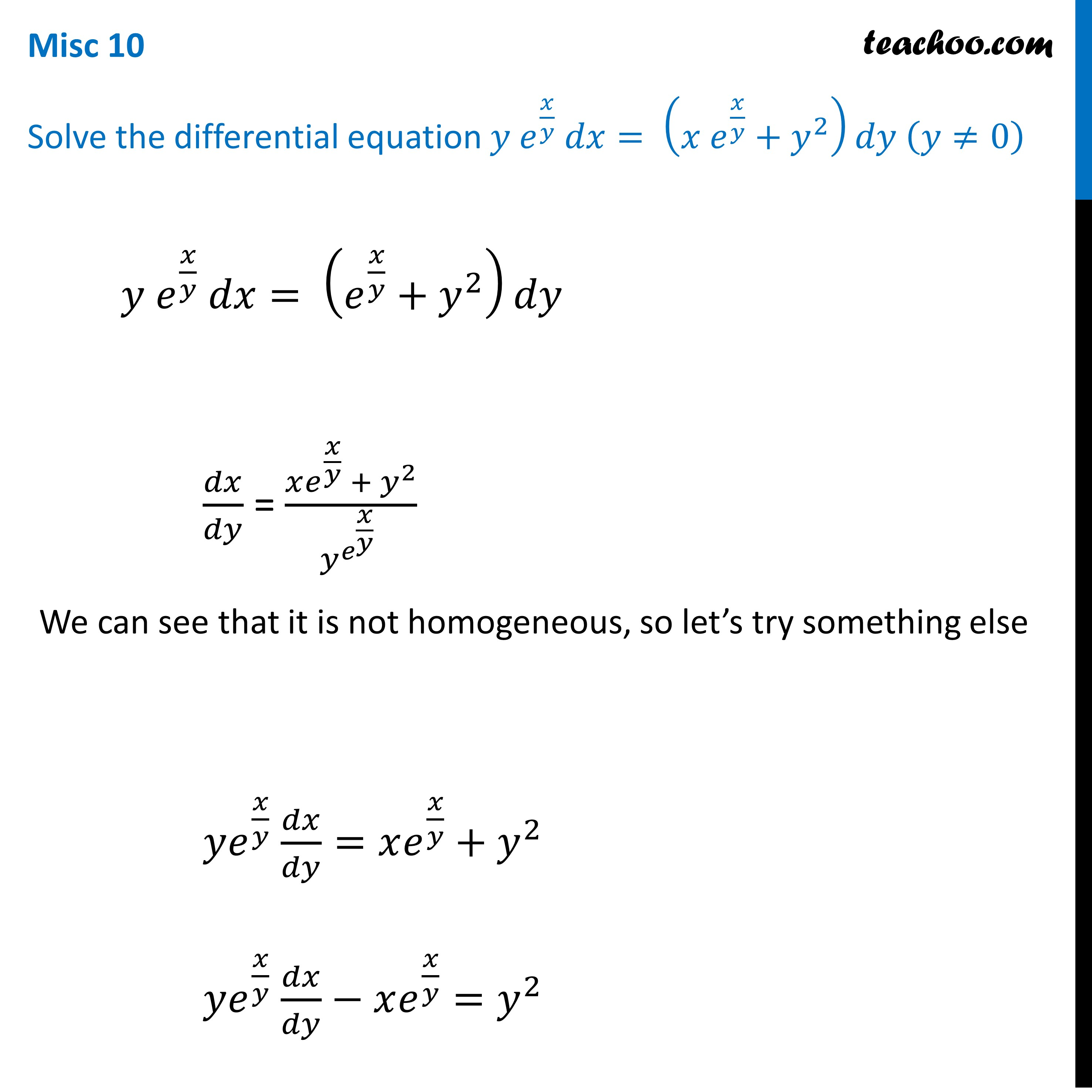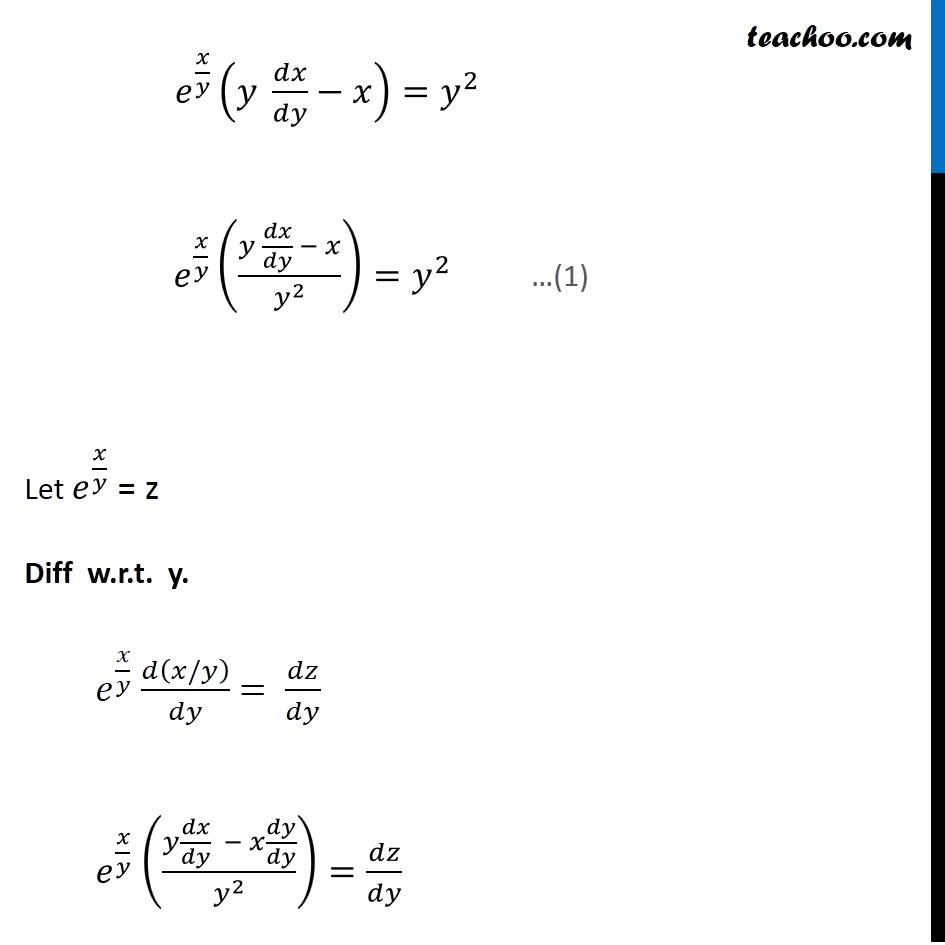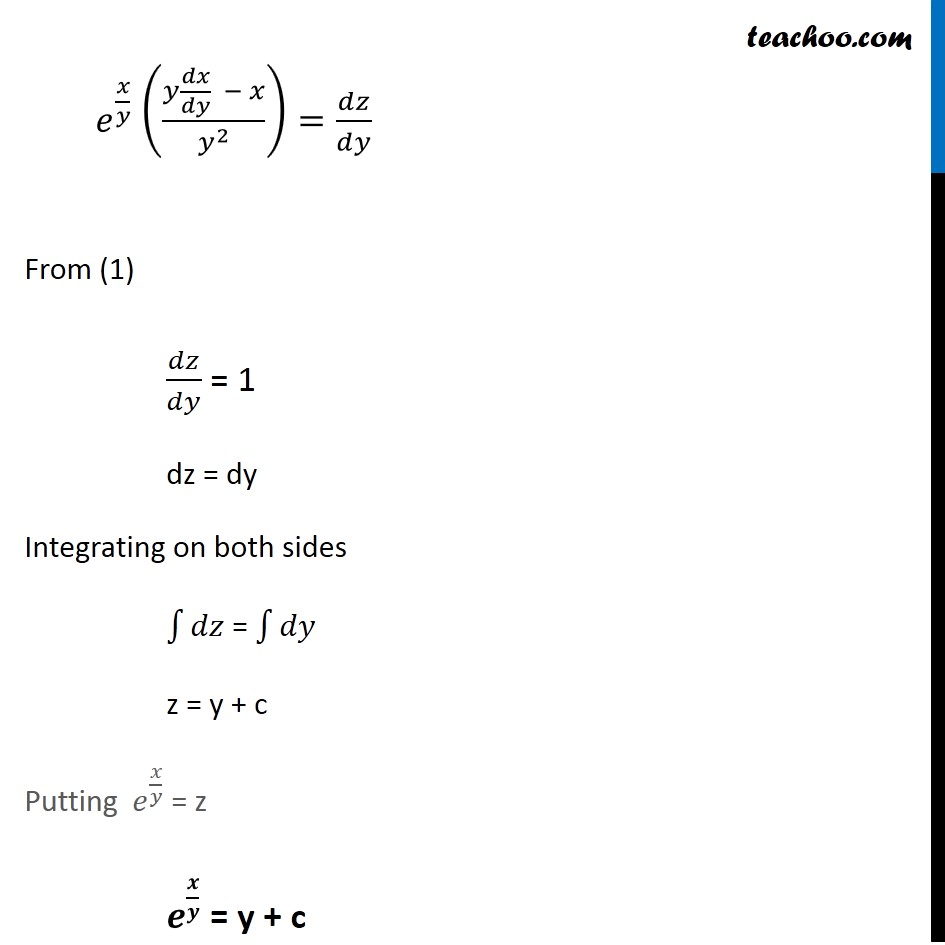Miscellaneous

Chapter 9 Class 12 Differential Equations
Serial order wiseLearn in your speed, with individual attention - Teachoo Maths 1-on-1 Class

### Transcript

Misc 8 Solve the differential equation 𝑦 𝑒^(𝑥/𝑦) 𝑑𝑥= (x𝑒^(𝑥/𝑦)+𝑦^2 )𝑑𝑦 (𝑦≠0) 𝑦 𝑒^(𝑥/𝑦) 𝑑𝑥= (𝑒^(𝑥/𝑦)+𝑦^2 )𝑑𝑦 𝑑𝑥/𝑑𝑦 = (𝑥𝑒^(𝑥/𝑦) + 𝑦^2)/(𝑦^(𝑒^(𝑥/𝑦) ) ) We can see that it is not homogeneous, so let’s try something else 𝑦𝑒^(𝑥/𝑦) 𝑑𝑥/𝑑𝑦=𝑥𝑒^(𝑥/𝑦)+𝑦^2 𝑦𝑒^(𝑥/𝑦) 𝑑𝑥/𝑑𝑦−𝑥𝑒^(𝑥/𝑦)=𝑦^2 𝑒^(𝑥/𝑦) (𝑦 𝑑𝑥/𝑑𝑦−𝑥)=𝑦^2 𝑒^(𝑥/𝑦) ((𝑦 𝑑𝑥/𝑑𝑦 − 𝑥)/𝑦^2 )=𝑦^2 Let 𝑒^(𝑥/𝑦) = z Diff w.r.t. y. 𝑒^(𝑥/𝑦) 𝑑(𝑥/𝑦)/𝑑𝑦 = 𝑑𝑧/𝑑𝑦 𝑒^(𝑥/𝑦) ((𝑦 𝑑𝑥/𝑑𝑦 − 𝑥 𝑑𝑦/𝑑𝑦)/𝑦^2 )=𝑑𝑧/𝑑𝑦 " " …(1) 𝑒^(𝑥/𝑦) ((𝑦 𝑑𝑥/𝑑𝑦 − 𝑥)/𝑦^2 )=𝑑𝑧/𝑑𝑦 " " From (1) 𝑑𝑧/𝑑𝑦 = 1 dz = dy Integrating on both sides ∫1▒𝑑𝑧 = ∫1▒𝑑𝑦 z = y + c Putting 𝑒^(𝑥/𝑦) = z 𝒆^(𝒙/𝒚) = y + c